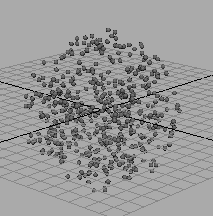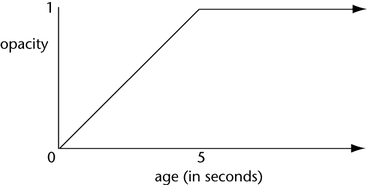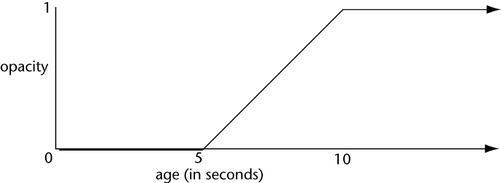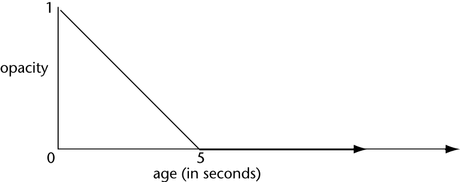# linstep

Returns a value from 0 to 1 that represents a parameter’s proportional distance between a minimum and maximum value. This function lets you increase an attribute such as Opacity from 0 to 1 linearly over a time range.

float linstep(float start, float end, float parameter)

start and end specifies the minimum and maximum values.

parameter is the value you want to use to generate the proportional number.

If parameter is less than start, linstep returns 0.

If parameter is greater than end, linstep returns 1.

Example

Suppose you’ve used the Particle Tool to create a collection of particles named Cloud:Suppose further you’ve added a dynamic per object Opacity attribute to Cloud (see Work with particle attributes). You then write this runtime expression for Cloud’s particle shape node:

```CloudShape.opacity = linstep(0,5,age);
```

This expression increases the per object Opacity attribute of CloudShape in equal steps from 0 to 1 for the first 5 seconds of the object’s existence. Because you created the object with the Particle Tool, the particles existence begins in the first frame of the animation.

All particles in the object fade in from transparent to opaque for the first 5 seconds of animation.

At the first frame that plays, the age of the particles is 0, so the linstep function returns 0 for the Opacity. An Opacity of 0 is transparent.

In each subsequent frame, the linstep function returns a proportionally larger opacity value. When the age of the object reaches 5, the linstep function returns 1 for the opacity. An opacity of 1 is 100% opaque.

When the age exceeds 5, the linstep function returns 1. The opacity stays 100% opaque. Here are some values returned for the object’s opacity:

Age Opacity
0.0417 0.0083
0.0833 0.0166
0.125 0.025
0.1667 0.0333
0.2083 0.0417
2.5 0.5
1.0 0.2
3.75 0.75
5 1
5.041 1
5.083 1
10 1

As the table shows, the opacity increases in linear increments for the first 5 seconds of the object’s age. At the midpoint of the specified 0 to 5 second age range, the opacity is 0.5. At 3/4 of the way between 0 and 5 seconds, the opacity is 0.75. At 5 seconds of the object’s age, opacity is 1. After 5 seconds, the opacity stays at 1.Suppose you edit the runtime expression as follows:

```CloudShape.opacity = linstep(5,10,age);
```

This increases the Opacity attribute linearly from 0 to 1 as the object’s age increases from 5 to 10 seconds.Suppose you edit the runtime expression as follows:

```particleShape1.opacity = 1-linstep(0,5,age);
```

This decreases the Opacity attribute linearly from 1 to 0 for the first 5 seconds of the object’s age. Subtracting linstep(0,5,age) from 1 causes the opacity to fade out rather than fade in.